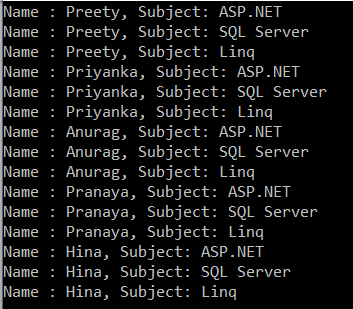# Linq Cross Join

## Linq Cross Join using Method and Query Syntax

In this article, I am going to discuss Linq Cross Join using both method and query syntax examples. Please read our previous article before proceeding to this article where we discussed Left Outer Join in Linq.

##### What is Linq Cross Join?

When combining two data sources (or you can two collections) using Linq Cross Join, then each element in the first data source (i.e. first collection) will be mapped with each and every element in the second data source (i.e. second collection). So, in simple words, we can say that the Cross Join produces the Cartesian Products of the collections or data sources involved in the join.

In Cross Join we don’t require the common key or property as the “on” keyword which is used to specify the Join Key is not required. And moreover, there is no filtering of data. So, the total number of elements in the resultant sequence will be the product of the two data sources involved in the join. If the first data source contains 5 elements and the second data source contains 3 elements then the resultant sequence will contain (5*3) 15 elements.

##### Model classes and Data Sources:

We are going to use the following Student and Subject model classes in this demo. Please create a class file and then copy and paste the following code in it.

```using System.Collections.Generic;

namespace LINQJoin
{
public class Student
{
public int ID { get; set; }
public string Name { get; set; }
public static List<Student> GetAllStudnets()
{
return new List<Student>()
{
new Student { ID = 1, Name = "Preety"},
new Student { ID = 2, Name = "Priyanka"},
new Student { ID = 3, Name = "Anurag"},
new Student { ID = 4, Name = "Pranaya"},
new Student { ID = 5, Name = "Hina"}
};
}
}

public class Subject
{
public int ID { get; set; }
public string SubjectName { get; set; }

public static List<Subject> GetAllSubjects()
{
return new List<Subject>()
{
new Subject { ID = 1, SubjectName = "ASP.NET"},
new Subject { ID = 2, SubjectName = "SQL Server" },
new Subject { ID = 5, SubjectName = "Linq"}
};
}
}
}```

As you can see we created two methods to return the respective data sources.

##### Example1: Cross Join Using Query Syntax

Cross Join Students with Subjects using Query Syntax.

```using System.Linq;
using System;
namespace LINQJoin
{
class Program
{
static void Main(string[] args)
{
var CrossJoinResult = from employee in Student.GetAllStudnets()
from subject in Subject.GetAllSubjects()
select new {
Name = employee.Name,
SubjectName = subject.SubjectName
};

foreach (var item in CrossJoinResult)
{
Console.WriteLine(\$"Name : {item.Name}, Subject: {item.SubjectName}");
}

}
}
}```

Output:We have 5 students in the student’s collection and 3 subjects in the subject’s collection. In the result set, we have 15 elements, i.e. the Cartesian product of the elements involved in the joins.

##### Example2: Cross Join using Method Syntax.

In order to implement the Cross Join using method syntax, we need to use either the SelectMany() method or the Join() method as shown in the below example.

```using System.Linq;
using System;
namespace LINQJoin
{
class Program
{
static void Main(string[] args)
{
var CrossJoinResult = Student.GetAllStudnets()
.SelectMany(sub => Subject.GetAllSubjects(),
(std, sub) => new
{
Name = std.Name,
SubjectName = sub.SubjectName
});

var CrossJoinResult2 = Student.GetAllStudnets()
.Join(Subject.GetAllSubjects(),
std => true,
sub => true,
(std, sub) => new
{
Name = std.Name,
SubjectName = sub.SubjectName
}
);

foreach (var item in CrossJoinResult2)
{
Console.WriteLine(\$"Name : {item.Name}, Subject: {item.SubjectName}");
}

}
}
}```

It will give you the same result as the previous example.

In the next article, I am going to discuss the Element Operators in Linq. In this article, I try to explain how to implement Linq Cross Join using both Method and Query Syntax with some examples.

### 2 thoughts on “Linq Cross Join”

1.Excellent example. Please correct spelling of Student

1.You missed a full-stop at the end 😉